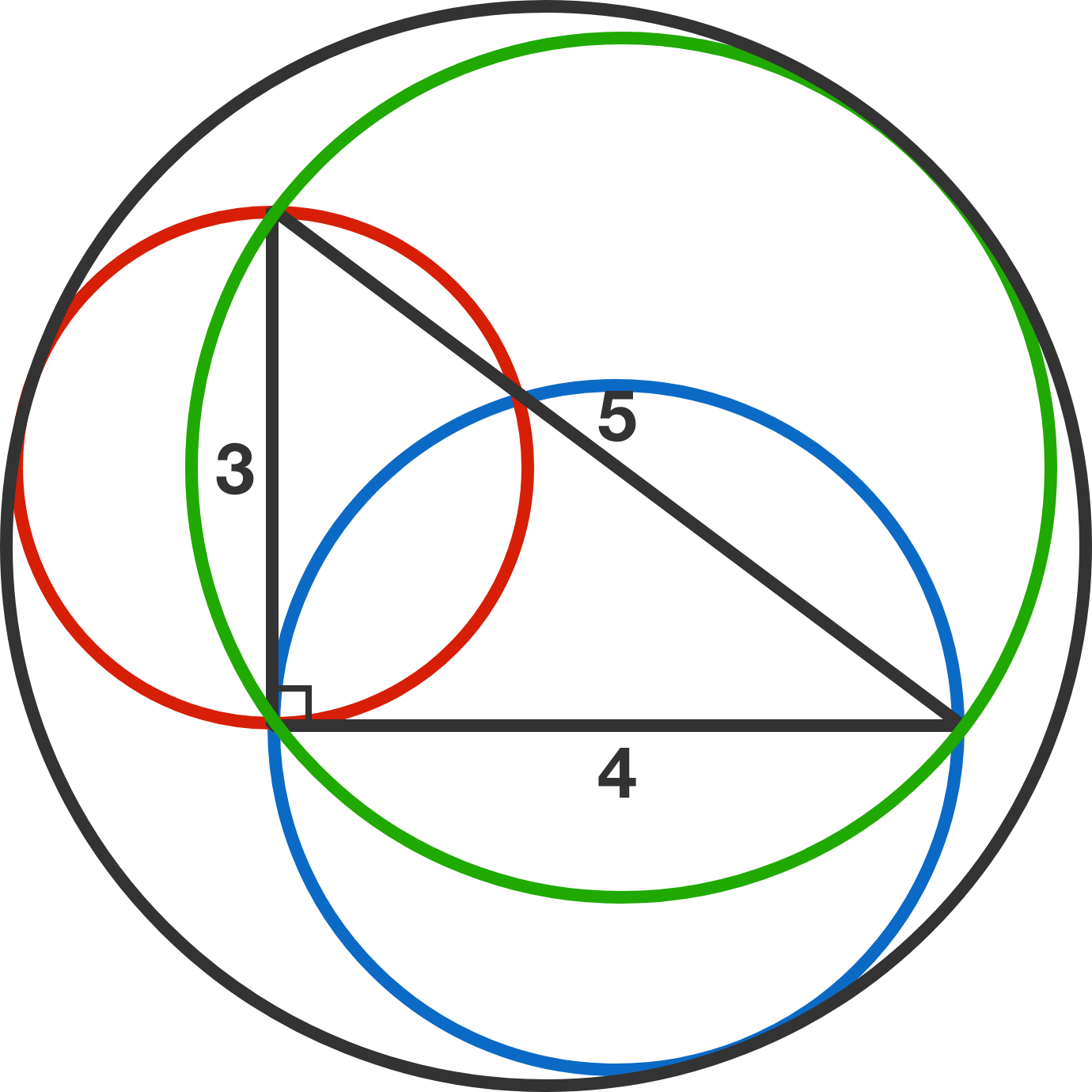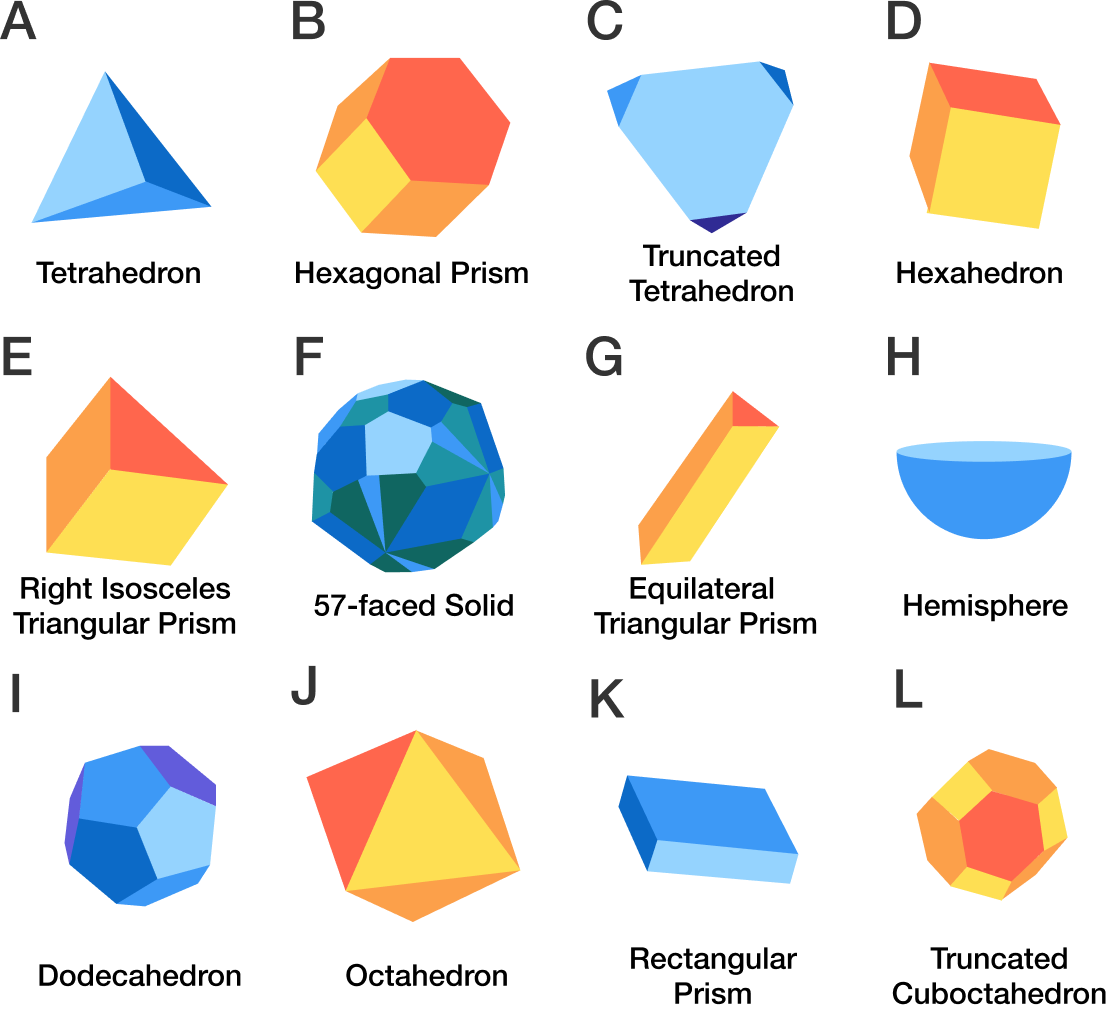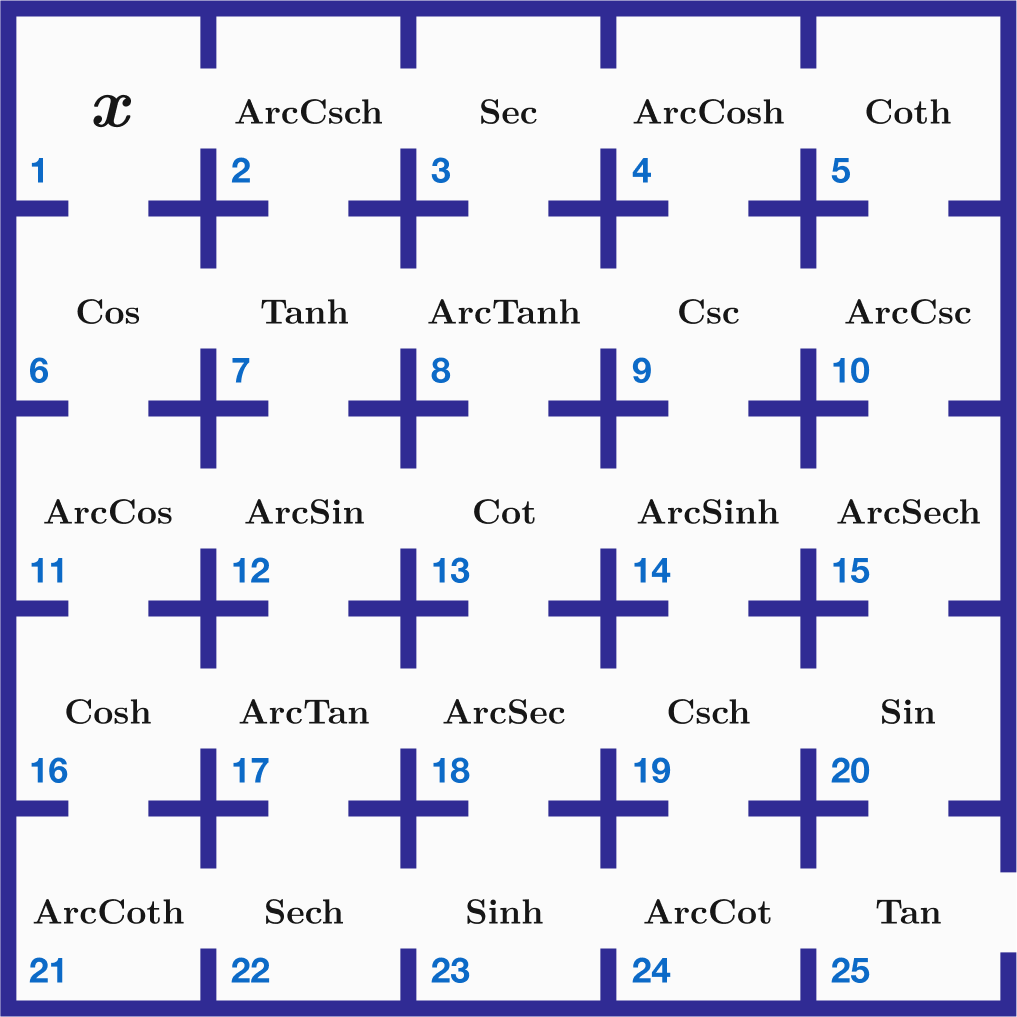# Problems of the Week

Contribute a problem

Can you color every positive integer either black or white such that there are no entirely white or entirely black non-constant infinite arithmetic progressions?This is not necessarily the coloring (if such a coloring is possible).

The red, blue, and green circles have diameters 3, 4, and 5, respectively.

What is the radius of the black circle tangent to all three of these circles (to 5 decimal places)?If you took a solid, 4-dimensional hypercube and cut it through with a single, flat, 3D hyperplane, which could not be the resulting cross-section?Select one or moreYou have to fill a large bag with 2018 fruits—apples, bananas, cantaloupes, durians, and raspberries—under the following restrictions:

• The number of apples must be a multiple of 41.
• The number of bananas must be even.
• There cannot be more than 40 cantaloupes.
• There cannot be more than one durian (for obvious reasons).

How many different ways are there to fill the bag?

Note: This problem can be solved without having to grind all the possibilities. Generating functions may help you.

You are in room $1$ in the upper left corner of a maze of rooms. The goal is to escape this maze and exit through room $25$ at the bottom right, but you can only move either right or down, passing through a total of $9$ rooms.

You need to deliberately find a path to get out exactly the same way you were when you started in room $1,$ because each room alters you, sometimes even making you imaginary.

What path will let you do that?Enter your answer as the total of the room numbers you must pass through in order to do that, a sum of $9$ numbers starting with $1$ and ending with $25$.

For example, if you go through rooms $1,2,3,4,5,10,15,20,25$, the total is $85$. However, you will experience the succession of functions
$\text{Tan}(\text{Sin}(\text{ArcSech}(\text{ArcCsc}(\text{Coth}(\text{ArcCosh}(\text{Sec}(\text{ArcCsch}(x)))))))))$ so that if you started with for example a real value of $0.5,$ you would end up being a totally imaginary number $0.714i$ when you leave the maze, which does not fulfill the requirement.

Note:

• $\large x$ cannot start with $i, -i$, or $0$ for this problem, but can be any other value, real or complex.
• See Gudermannian Function.
×